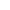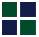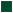Vedic Mathematics Made Easy``` Addition:Vedic Mathematics does not have techniques to help with addition unlike multiplication, division or factorisation, though it provides some interesting checking methods. Vedic Mathematics does not talk about simplified addition. However it does provide an interesting way of checking the result for any addition via technique called the ??Digit Sums Check??. To understand this technique please view the following movie: ``` Vedic Math Digit Sums Check ```Now using the Digit Sums Check please try to check if the answers for the following additions are correct: 3456 --> 3+4+5+6 = 18 --> 1+8 = 9 + 7687 --> 7+6+8+7 = 28 --> 2+8 = 10 --> 1 _______ 11143 --> 1+1+1+4+3 = 10 --> 1 i.e. 9+1 = 10 --> 1 So sum is correct. Note this technique is not full proof in the sense that if someone wrote the answer as 18343 the sum of digits would come equal to 1. But this is not the correct answer. However the method can be used for a quick second check of the result. Also small careless mistakes can be easily detected by this method. Try some more checks: 1) 3455 2) 75668 3) 867777 +6475 + 6576 + 898989 ______ _______ ________ 9940 82244 1766766 Answers: 1 is incorrect and 2 and 3 are correct. If you are as impatient as me and you honestly tried solving the problems above, you would be saying well the checking is so cumbersome that I need to check the check result!!! Well Vedic Maths is all about simplicity. So obviously the Check Digits is not complete without discussing the "Casting Out of Nines" method. Please take a look at the movie below to appreciate this technique. ``` Vedic Math Digit Sums & Casting Out the Nines Vedic Math: 9 Point Circle ``` Now try the same problems that we had struggled with earlier: 3456 --> 3+4+5+6 --> 9 + 7687 --> 7+6+8+7 = 7+2+8+1+7+2+1 --> 1 (Note to make 9s I split the number 6 to 2+1+2+1. With little practice you can do this mentally) _______ 11143 --> 1+1+1+4+2+1 --> 1 i.e. 9+1 = 10 --> 1 Now retry the following examples: 1) 3455 2) 75668 3) 867777 +6475 + 6576 + 898989 ______ _______ ________ 9940 82244 1766766 Does it simplify things? Take a break now and let your brain sub-conciously absorb what you have read till now. It has been found that long term memory is increased if you read stuff with a gap, i.e you read something and then come back to the same topic again after some time then your retention of the concept is for a longer term. ``` ```Hope you had an interesting break!! Please try to recollect all that you had read in the earlier sections...The Digit Sum Check, the Casting out of nines technique. Now try to check some more addition examples. 1) 96789 2) 78668 3) 67777 +45621 + 86576 + 98989 ______ _______ ________ 142410 165244 165766 4) 9622 5) 23567 6) 87393 +6475 + 12332 + 12238 ______ _______ ________ 16097 37899 99631 7) 88992 8) 46804 9) 2468 +12344 + 23676 + 2009 ______ _______ ________ 101336 70483 4479 Did you get the wrong answers. Would it have helped if you had this problem in the office or in the school to quickly check the results of your calculation. Wrong Answers in the above cases: 3, 5, 8, 9 ```The Cosmic Science My Favorite Links: Home The 16 Sutras Subtraction Multiplication